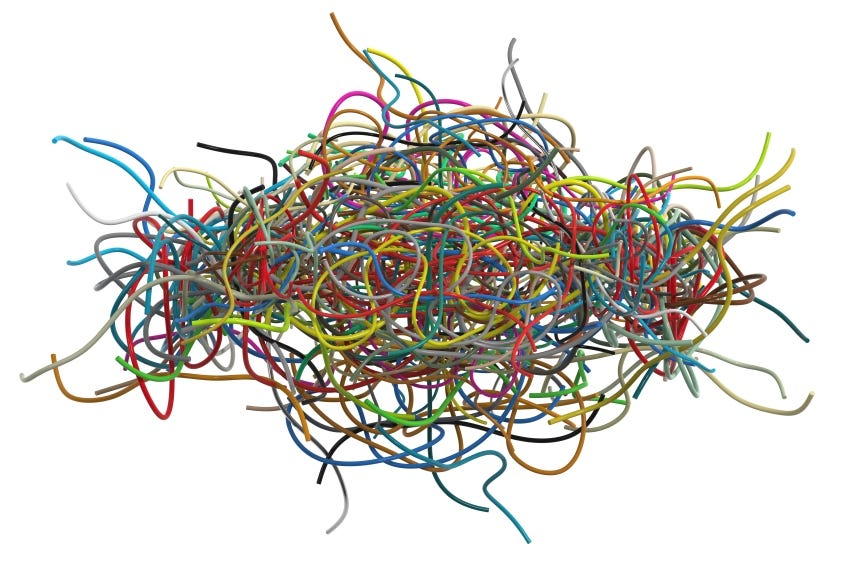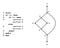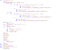# Data Processing Programming, a Software Engineering approach (2): Code Complexity

## For programmers, data engineers, data scientists and anyone who programs on dataPhoto from BANKING HUB

# Part I: Conceptual Framework

I think instead of opening a new subject by giving a bunch of definitions, it is more useful to develop it, going through the thought process together with the reader or audience (In general one has to be very careful with definitions, for they can be useful when used properly but in many other cases they can work as inhibitors for a subject exposition, since by setting the boundaries for a problem, at once limit their expansion. Anyways, this is a question in theory of definitions in philosophy and we will not dwell on it here.)

So, let’s begin it. When programming, the right way is to think about it, to look at it from another person’s perspective. This idea can be stated as the following principle:

Write the code such that for someone else, completely unfamiliar with it, it should require the least amount of effort to understand it.

This means, to put the same meaning in other words, programmer should intend for simplicity or program should be simple. Thus, simplicity is the first concept so far encountered in trying to formulate a conceptual framework for Data-processing programming (DPP). For now, there is no proof given for the above statement and let’s accept it as an axiom. It will be justified later, throughout this writing.

Okay, then what is simplicity? It turns out that defining simplicity directly is not easy (without stating the same thing in other words) nor is it that useful to do so. Instead, a better approach would be to understand it through its opposite — complexity. Because, at least in the context of coding as it will be shown, simplicity is nothing but avoiding complexity. Avoiding complexity though is possible only by understanding it. Now that we have the second important concept in our investigation of the subject of programming — complexity — let’s understand what it is.

# What is Complexity?

## It is NOT about Computational Complexity

Computational complexity is not of interest to us and is not our subject of discussion. As was stated earlier, computational complexity is a runtime measure, runtime behavior of algorithms and which is its fundamental property. Instead, we are interested in the complexity of the code of the program. From this point onward, we will completely forget about computational complexity.

Now let’s move on to the next topic, cyclomatic complexity.

## Cyclomatic Complexity

Cyclomatic complexity is a software metric used to indicate the complexity of a program. It is a quantitative measure of the number of linearly independent paths through a program’s source code.

To put it simply, based on this metric, for a piece of code, the more possible paths of execution, the more complex it is. For example, if the following is the whole code under consideration,

`z = x * 2`

its complexity 1. Since there is only one path for this code. But for the following code complexity is increased, it is now 2

`if (condition is true) then    z = x * 2else    z = x * 4`

Because, depending whether the condition evaluates to true or false, there are two paths along which the program can run. Thus, the second program is more complex than the first one.Image showing code and it’s corresponding cyclomatic graphPhoto from Craftofcoding

The concept of cyclomatic complexity is useful as it provides an insight into the nature of code complexity. Any serious programmer has to know it. Yet, as important as it is, it is not broad enough and does not explain the types of complexity that commonly arises in data-processing programming. Why? Because it is focused on measuring the control flow of the program. Control flow analysis, while an important factor in programming paradigms, in DPP many of computations are declarative in nature (like in SQL) where there is no control flow and their complexity comes from other sources. Even in most cases of if-else, switch or any conditional statements, their semantic can be recast so it becomes declarative, eliminating control flow concern (this will be a major topic in part III). To understand the type of complexity associated with DPP, a new concept which I call structural complexity is introduced.

## Structural complexity

In mathematics, linear equations are the simplest of all equation types. It is the simplest because it is the easiest to understand and to solve. That’s why it is the first type of equation one learns at school. (Note: Equations and examples are intentionally simple, only to make the point and not to take us away from focusing on our subject of inquiry.)

Anyways, the following is a linear equation:

`24 = 2x                                     (1)`

Easy to solve, isn’t it? Just divide both sides by 2 and the solution is x = 12. Now, consider the next equation.

`24 = 2x + 5x — 3x + 6x + 4x                 (2)`

This equation appears more complicated than the first one; it is longer; has more terms etc. But, it is not. It can be reduced to

`24 = 20x`

Therefore, in spite of its more complicated-looking view, in terms of complexity it is exactly equal to the first equation. In other words, they have the same degree of complexity.

Now consider the following quadratic equation:

`24 = 2x^2                                     (3)`

Although it looks simpler (shorter and more compact) than equation (2), in fact it is of a higher degree of complexity. It is not as intuitive and easy to understand as the linear equation. It can no longer be solved with the means of basic arithmetic operations `(+, -, *, / )`. It requires a separate method (Quadratic formula) and more mathematical devices such as radicals, irrational numbers, multiple solutions etc (In fact, modern formulation of quadratic formula had to wait until 1637 by Rene Descartes.) Speaking in terms of structural complexity `(2x^2)` is a more complex structure than `(2x + 5x — 3x + 6x + 4x)` as it is more difficult to understand and to solve.

Taking this analogy to the field of programming, the following nested loop

`for i in array1:    for j in array2:        Do something involving i and j at once`

is more complex than the next code snippet consisting of two loops.

`for i in array1:    Do something with ifor j in array2:    Do something with j`

Why? Because in the first case, one needs to trace the values of indices, i and j, together with all variables and logic associated with them at once, as both loops progress (it gets more complicated as the loops and the operations within them get more complex). The two loops are intertwined and one cannot be separated from the other. While in the second case, each loop is independent of the other and it involves only tracing progression of one at a time. Hence simpler!

Now let’s take this idea one step further, or, one step closer to our subject of study, an example from data-processing programming. A nested query, like the following

involving three layers of SELECT, is more complex than three SELECT queries in sequence.

As the last example to reinforce the notion of structural code complexity, now we know the the following code is complex and why so.Code example from Quora

Structurally more complex code involves more dimensions to consider at once, hence it can also be called dimensional complexity.

Emphasis: here, it is not being suggested that things like nested loops or nested queries are always bad and to be avoided; it would be naive to say so.

In the same way that in mathematics equations of different degrees and types are necessary, these constructs are part of programming and exist for a reason. The problem is not with the language constructs themselves but in how they are used. In later parts, these cases will be contextualized. Here they are used only to illustrate the idea of structural code complexity.

<< Previous : Data Processing Programming, a Software Engineering approach(1): Introduction

Next: Data Processing Programming: a Software Engineering Approach (3): Separation of Concerns >>

Originally published at https://objectacademy.com.